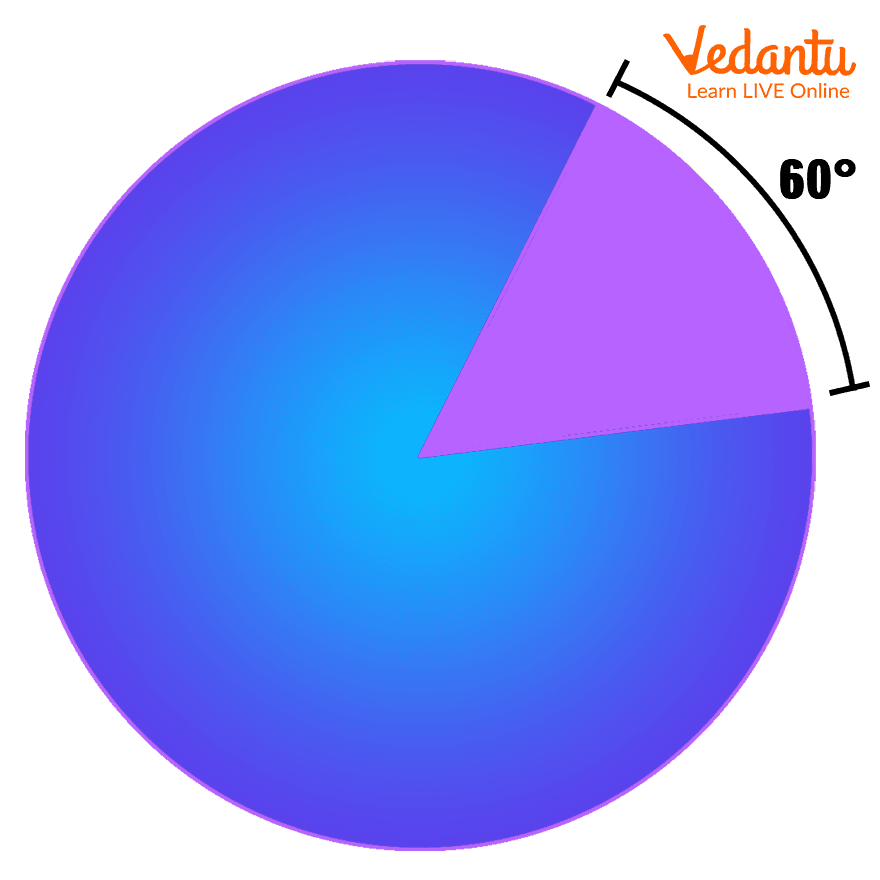Courses
Courses for Kids
Free study material
Free LIVE classes
More

# Degree - Definition, Symbol, and Degree to Radian ConversionLIVE
Join Vedantu’s FREE Mastercalss

## What is ‘Degree’?

How far is your school from your home? How much time do you take to reach your home? 10 km, and it will take 15 minutes by bicycle. And, how far is your best friend’s home from yours and how much time do you take to reach his home? Approximately 500 metres, and 2 to 3 minutes by walk. What do km or metre and minutes represent here? These are the units of distance and time, respectively. Do you know what is the unit to measure an angle?

The unit which is used to measure the angle is ‘degree’. In this article, we will dive deep into the definition of ‘degree’, its symbol, and its conversion into another unit. So waiting for what my dear child, let us go!

### Definition of Degree

In Geometry, the unit which is used to measure the angle is known as 'degree'. There are other units also used to measure the angle, but the degree is most popular and commonly used amongst all. The other common unit used to measure the angle is ‘radian’. It is the SI unit to measure an angle. We can convert both units into one another using some formulas.

A protractor is used to measure the angles in degrees.An example of ‘degree’; degree measure 60

### Degree Symbol

A degree is represented by the symbol °. It is of the shape of a small circle that would be written after a number and on its top such as 0 degree = 0°, 90 degree = 90°, 180 degree = 180°, etc.

### Types of Angles on the Basis of Their Degrees

There are different types of angles based on their measurement. Some of them are listed below:

Acute Angle: The angle which measures more than 0° and less than 90°, is called an acute angle.

Right Angle: The angle which measures 90° is called the right angle.

Obtuse Angle: The angle which measures more than 90° and less than 180°, is called the obtuse angle.

Straight Angle: The angle which measures exactly 180° is called a straight angle.

Reflex Angle: The angle which measures more than 180° and less than 360° is called a reflex angle.

Complete Angle: The angle which measures 360° is called a complete angle. A complete circle measures 360°.

### Conversion of Units of Angle

There are mostly two units used to measure the angle, radian and degree. They both are interchangeable. Now let us see how to convert both of them.

To convert degree to radian, we use the following formula:

Radian = $\frac{angle \, in \, degree\times \Pi }{180^{0}}$

To convert radian to a degree, we use the following formula:

Degree = $\frac{angle \, in \, radians \times 180^{0}}{\Pi}$

### Numerical Type Questions

Solution: To convert degree to radian, we can calculate as:

Angle in radian = $\frac{angle \, in \, degree \times π}{180^{0}}$

= $\frac{45^{0} \times \Pi}{180^{0}}$

= $\frac{\Pi}{4}$

2. A child measures an angle of 2𝜋 radian. Convert it to the degree.

Solution: To convert radian to degree, we can calculate as:

Angle in degree = $\frac{angle \, in \, radians \times 180^{0}}{\Pi}$

= $\frac{2\Pi \times 180^{0}}{\Pi}$

= $180^{0}$

### Points to Remember

1. If θ is the measured angle, then we can remember the names of the angles and their measurement as:

 Measurement of Angle Name of Angle 0°⩽θ⩽90° Acute angle θ=90° Right angle 90°⩽θ⩽180° Obtuse angle θ=180° Straight angle 180°⩽θ⩽360° Reflex angle θ=180° Complete angle

2. A protector can measure the angle in two ways:

• Clockwise direction

• Anti-clockwise angle

Last updated date: 27th Sep 2023
Total views: 138k
Views today: 3.38k

## FAQs on Degree - Definition, Symbol, and Degree to Radian Conversion

1. What is the degree? What are its applications?

‘Degree’ is a unit to measure an angle in geometry. It is most often used in daily life to measure an angle. In construction, designing, technology, and in many other fields, the angle is measured in degree. It makes the calculation very easy.

2. What are the different types of angles?

There are different types of angles such as acute angle, right angle, obtuse angle, straight angle, reflex angle, complete angle, etc. An angle measuring exactly 90°, 180°, and 360° is called right angle, straight angle, and reflex angle, respectively. An angle that is less than 90° and more than 0° is called acute angle whereas an angle that measures more than 90° and less than 180° is called obtuse angle. Also, if an angle measures more than 180° and less than 360° is called a reflex angle.